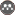Volume 22, Issue 4 (Winter 2019)                   JWSS 2019, 22(4): 279-290 | Back to browse issues page

BibTeX | RIS | EndNote | Medlars | ProCite | Reference Manager | RefWorks
Send citation to:Majedi Asl M, Fuladipanah M. Application of the Evolutionary Methods in Determining the Discharge Coefficient of Triangular Labyrinth Weirs. JWSS. 2019; 22 (4) :279-290
URL: http://jstnar.iut.ac.ir/article-1-3586-en.html
1. Department of Civil Engineering, Faculty of Engineering, University of Maragheh, Maragheh, Iran.
Abstract:   (748 Views)
A labyrinth weir is a nonlinear weir folded in the plan-view which increases the crest length and the flow rate for a given channel width and an upstream flow depth. Nowadays, a labyrinth weir is an attractive alternative for those weirs that have a problem in passing the probable maximum flood. The three-dimensional flow pattern and unlimited geometric parameters provide a major challenge to the designers of these weirs. The present study aimed at determining discharge coefficients of sharp-crested triangular labyrinth weirs using the support vector machine (SVM). The results were compared with the experimental data. For this purpose, 123 laboratory test data including  geometric and hydraulic parameters such as vertex angle (θ), magnification ratio (L/B), head water ratio (h/w), Froude number (Fr), Weber Number (We) and Reynolds number (Re) were used. The results showed that the SVM-based model produced the most accurate results when only three geometric parameters, e.g. (h/w, θ, L/B), were introduced as the input parameters (R2 = 0.974, Root mean square error [RMSE] = 0.0118, mean absolute error [MAE] =0.0112 and mean normal error [MNE] =0.017 for the test stage). Also, for these weirs, polynomials linear and nonlinear regression equations were presented. Finally, the discharge coefficient of sharp-crested triangular labyrinth weirs based on the Rehbock equation was evaluated and compared with the SVM using nonlinear and linear regression methods.